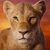# Are You A Mental Math Genius? Solve These Difficult Math Problems

## Only super math geniuses can quickly solve these mental math problems. And here we go!Created by Lea Abelson
On Apr 1, 2022
1 / 10

5 X 6 =

2 / 10

70 X 12 =

3 / 10

78 X 40 =

4 / 10

56 + 9 =

5 / 10

87 - 45 =

6 / 10

112 X 56 =

7 / 10

98 + 56 =

8 / 10

43 / 112 =

9 / 10

88 / 5 =

10 / 10

600 - 48 =

10
Questions left
These are 10 of the World CRAZIEST Ice Cream Flavors
Created by Tal Garner
On Nov 18, 2021